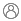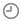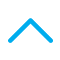Contact usTo Buy: 1800-258-5899 (9:30 AM to 6:30 PM)

|For Existing Policy: 1800-103-0003/ 1800-180-0003/ 1800-891-0003

|customerservice@canarahsbclife.in

|Locate Branch

|Login# Internal Rate of Return: Meaning, Formula and UsageKnowledge Centre TeamOctober 07, 2022178 Views

There are a variety of ways to measure the performance of an investment. Some common methods include calculating the return on investment (ROI), net present value (NPV), and internal rate of return (IRR). What is IRR and how is it different from others and when is it typically used?

## What is the Internal Rate of Return (IRR)?

The Internal Rate of Return (IRR) is a metric used in capital budgeting to estimate the expected return of an investment. The IRR is the discount rate that makes the net present value of an investment equal to zero.

The IRR is a good way to compare different investments. It is also a good way to compare different projects within the same company. Higher the IRR, the higher the profit from the investment.

### Example of IRR Calculation

Assume a project has an initial investment of Rs 1,000 and is expected to generate cash flows of Rs 200, Rs 300, and Rs 400 over the next three years.

The project's IRR would be calculated as follows: IRR = [Rs 200 + Rs 300 + Rs 400] / [3 * Rs 1,000] = 0.14 In this example, the project has an IRR of 14%.

You should use the internal rate of return (IRR) when you are trying to compare different investments. The IRR is a good way to measure the return of an investment, as it considers the timing of the cash flows.

## How to Calculate IRR?

There are quite a few methods to calculate IRR:

1. One popular method is to use a financial calculator, which can be found online.

2. Another method is to use Excel, which has a built-in function for calculating IRR.

3. Use the conventional formula on paper

Using the Financial Calculator

1. Enter the cash flows for the investment, making sure to list them in chronological order.

2. Press the "IRR" button on the calculator.

3. Enter the required rate of return. This is the minimum return you would accept for investing

4. Press the "Calculate" button.

5. The Output will show the Internal Rate of Return for the investment.

Using MS Excel

1. Enter your investment's cash flows in a table. Make sure to list all cash flows in chronological order and label the table appropriately

2. Determine the number of periods for your investment. This is the number of cash flows you have listed in your table

3. Calculate the interest rate per period. This is simply the interest rate divided by the number of periods

4. Use the IRR function in Excel to calculate the internal rate of return for your investment

A positive IRR indicates that your investment will generate a return greater than the interest rate, while a negative IRR indicates that your investment will generate a return less than the interest rate.

Using Math Formula

1. Step 1: Arrange the cash flow in increasing order, i.e., negative values first followed by positive values.

2. Step 2: Calculate the cumulative cash flow.

3. Step 3: Use the following formula to calculate the IRR

IRR = [∑(CFn * (1 + r)n)]/∑(CFn)

Where,

CFn = Cash flow for period n
r = Rate of return
n = Number of cash flows

## How to Use IRR for Investments?

IRR, or internal rate of return, is a financial metric used to assess the profitability of investments. To calculate IRR, the investor must first determine the cash flows associated with the investment. These cash flows can be positive (inflows) or negative (outflows).

Once the cash flows have been determined, the investor must then calculate the IRR. The IRR is the rate at which the present value of the investment's cash flows equals the initial investment. In other words, it is the discount rate that makes the net present value of the investment equal to zero.

The higher the IRR, the more profitable the investment. For this reason, investors often use IRR to compare different investment opportunities.

Recommended Reading - What are Financial Assets?

## When Not to Use IRR?

The internal rate of return (IRR) is a good measure to use when comparing investment opportunities, but there are some potential drawbacks.

• The biggest issue with using IRR is that it assumes that cash flows are reinvested at the same rate. This may not hold good in the real world.
• Another issue is that IRR does not consider the time value of money. This means that it does not consider the fact that money today is worth more than money in the future.
• Finally, IRR can give conflicting results when there are multiple periods of positive and negative cash flows.

## Internal Rate of Return Vs Net Present Value

IRR and NPV are two methods of investment appraisal.

 IRR NPV IRR indicates the discount rate at which the NPV of a project is zero. NPV is the present value of cash inflows from a project minus the present value of cash outflows. IRR is a measure of the expected return on investment. NPV is a measure of the profitability of an investment. IRR shows the percentage return that the project will yield NPV shows money that a project will produce IRR is focused on breaking even on cash flow NPV is focused on project surplus

## Investments Where IRR Helps to Judge Performance

IRR is a useful tool to judge your investments with regular cash inflows or outflows. So, you can use IRR to estimate your returns from all the SIP investments or regular income plans like:

- Mutual fund SIPs
- Unit Linked Insurance Plans (ULIPs)
- Monthly Income Plans (or IDCW Mutual Funds)
- National Pension Scheme (NPS)

IRR will help you estimate the actual return on your annuity or SIP which you can compare to lump sum investments or other investments declaring annual CAGR growth.

Do remember to convert your monthly IRR to an annual rate of return before you can compare it with CAGR or compounded annual growth rate.

You should also consider the different scenarios and components of the project. You must have a firm understanding of the risks involved in the project and the probability of success of the project. For more clarity on the potential impact of the project on your business, you should use multiple methods, to calculate the return on investment, before deciding.

Disclaimer: This article is issued in the general public interest and meant for general information purposes only. Readers are advised to exercise their caution and not to rely on the contents of the article as conclusive in nature. Readers should research further or consult an expert in this regard.

### Browse by Categories##### Try OurCalculators### Get a Call Back

Do you want us to call back Please fill the form belowAnnual Income (In Lacs)### Our ProductsTerm Insurance Plan

Life Cover till 99 years of age

Option to Block the premium rate and increase cover by upto 100% at the blocked rate

Option to avail monthly income post attaining 60 years of age

Tax Benefits as per applicable lawsSavings Plan

Better value for high premium commitment

Guaranteed benefits payable on maturity

Life cover for the entire term

Flexibility to choose premium payment termsSAVINGS PLAN

5 plan options to choose from to protect your loved ones

Pay premiums for 5,7, or 10 years as per your financial goalsCall BackPay Premium